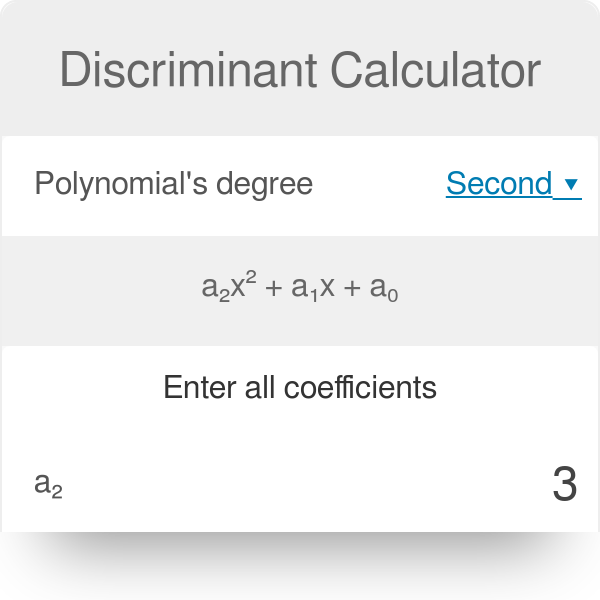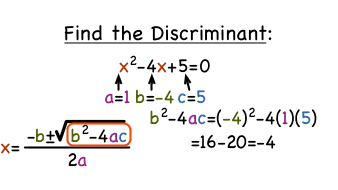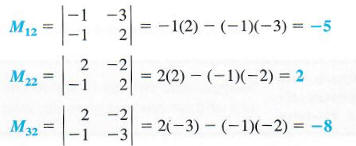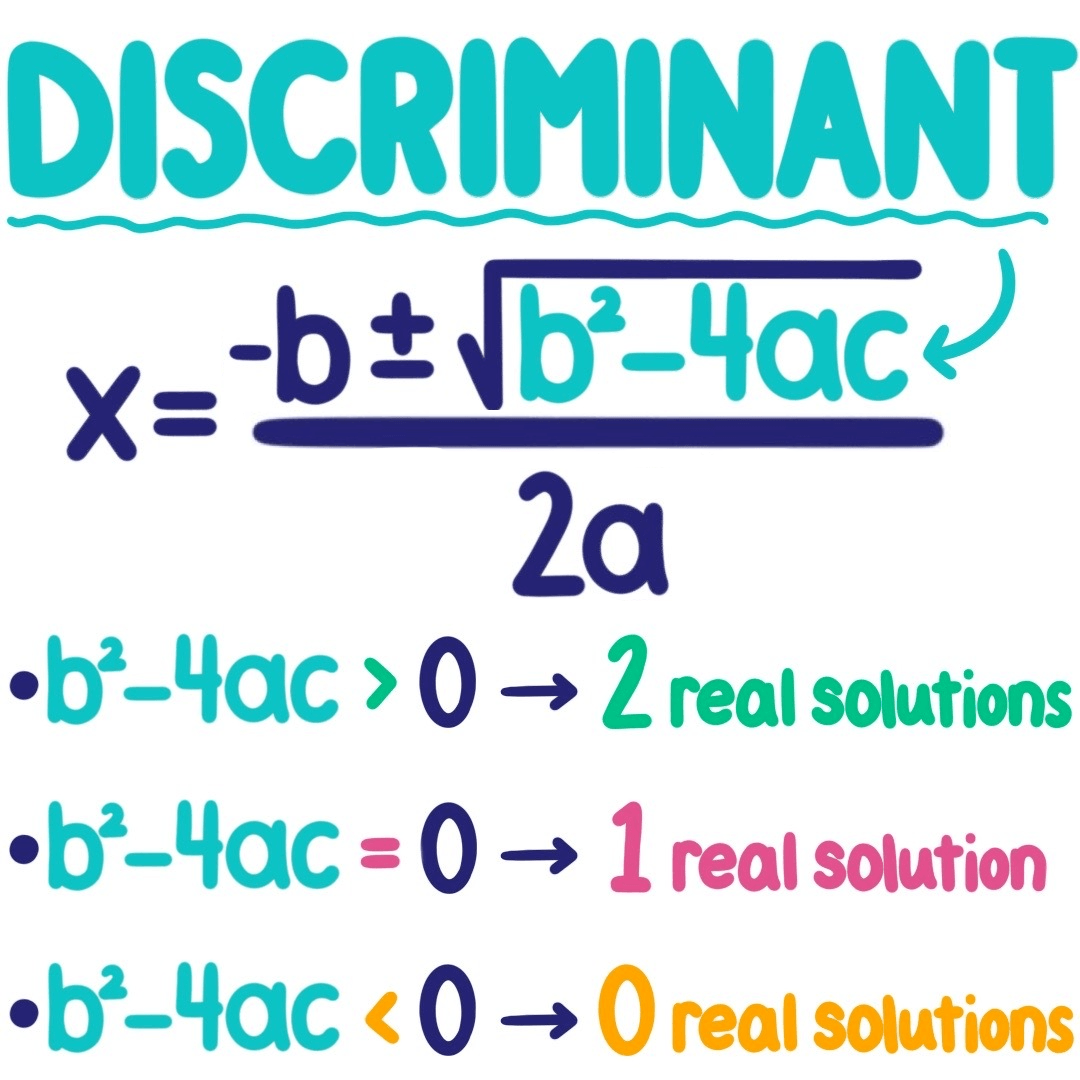Thursday, 19 May 2022

# Discriminant Calculator: Definition, equation

The discriminant calculator is a free online app. However, It calculates the discriminant value of quadratic equation coefficients. An online discriminant calculator tool speeds up and simplifies computations. Also, it displays the answer in a fraction of a second. In this article, we are talking about this calculator. So, please keep reading to know more about it.## Discriminant calculator definition

A discriminant is a function of a polynomial equation’s coefficients that expresses the nature of the roots of a particular quadratic equation. However, the equations may distinguish between many sorts of answers, such as:

• When the discriminant value is positive, we have two distinct solutions.
• When the discriminant value is zero, there is only one true solution.
• Also, we get a pair of complicated solutions when the discriminant value is negative.

The discriminant calculator is a free online calculator that computes the discriminant of a given quadratic equation. Then, for a quadratic equation ax2 + bx + c = 0, where a is not equal to 0, the discriminant formula is b2 – 4ac.

Discriminant is also represented by the sign.

Hence, Δ = b2 – 4ac

However, the discriminant value aids in decoding.

Moreover, the number of roots determines whether the quadratic equation has two roots, one root, or none at all. The type of roots determines whether the roots are real or imaginary.

## Discriminant calculator with steps

The following is how to use the discriminant calculator:• Enter the coefficient values “a”, “b”, and “c” into the appropriate input areas.
• To obtain the results, click the “Solve” button.
• In the output field, the discriminant value will be presented.

## Discriminant calculator with k

Discriminant equals b squared minus 4ac. We will know that there are three cases that are possible for the discriminant.

So, in the first case, we have a positive discriminant. It is greater than zero.

• b square minus 4ac greater than zero.

Then, in the second case, we have exactly zero for our discriminant.

• b square minus 4ac equals zero.

Then, in the third case, we have a negative discriminant.

• b square minus 4ac less than zero.

### First case

So, the first case, if we have a positive because we have something to add and subtract. Then, we are gonna have two roots, remember adding, subtracting and coming from the axis of symmetry. They give us two different x-intercepts.

### Second case

So, the second case is the very specific case where you only get one real root. Because your root vertex is right on the x axis. It is the x intercept.

### Third case

So, the last case is when you try to square root a negative, you get zero real roots. It means you actually don’t have any x intercepts.

We are gonna make use of these three cases to solve three different questions.

So, the suggestion is firstly recognize which equation is which case. Usually if you have to do all of them, do one real root first. Also, when we do one real root, all that means the discriminant has to be equal to zero. So, we don’t have to worry about inequalities, we are using equal signs. We can set the discriminant to zero.We will say a is 1, b is K and I changed the c value to positive 9. Once we plug our values in, we square root for K. We get plus or minus 6. Actually we are doing a perfect square trinomial. It means if we were to graph it, our vertex in each of those cases would either be positive 3 or negative 3. It means we had been right on the x axis. So, the vertex is the x intercept. In the particular case, where we have one real root.

### Example 1

b square minus 4ac greater than 0

So, the answer is if K is plus or minus six, we have to set a discriminant to be positive. So, basically K has to be greater than six. Or, we could say that K is less than negative six. Because when we square negative seven or we square negative eight, it’s still going to be greater than 36. So, as long as we are outside of the sixes we are fine.

### Example 2

b square minus 4ac less than zero

The last case is when we have no real roots or we have a negative discriminant, It’s just sort of the opposite of what we just did. However, it is in between negative six and six as long as its absolute value is in between six and negative six. Then, we will have no real roots.

## Discriminant Calculator with step by steps

### Step 1

The first step in using Discriminant Calculator is to transform the quadratic equation where we want to apply Discriminant Calculator into Standard Form of Quadratic Equation.

However, ax^2 + bx + c = 0 is the standard form of a quadratic equation.

As a result, the first step is to write our quadratic equation in standard form, which is ax^2 + bx + c = 0.

Assume we need to solve a quadratic equation that is now in the form of

-16 = x^2 – 10xSo we rearrange this equation to give it the conventional form ax^2 + bx + c = 0. Hence,

x^2 – 10x = -16 becomes x^2 – 10x + 16= 0.

The quadratic equation has now been transformed into standard form.

### Step 2

This is the most significant stage, in which the values of coefficients a, b, and c are determined by comparing them to the Standard Form of Quadratic Equation ax^2 + bx + c = 0.

For example, when we compare x^2 – 10x + 16= 0 to ax^2 + bx + c = 0, we obtain

a = 1; b = -10; and c = 16

### Step 3

Enter the values of a, b, and c into the discriminant calculator formula b^2 – 4ac.

As a result, Discriminant, D = (-10) 4(1)(16) – 2 = 36

Because D > 0, the equation has two unique and actual roots.

## Discriminant Calculator cube

A polynomial’s discriminant is a function of its coefficients in algebra. It describes the characteristics of its roots. Discriminant is frequently abbreviated as ‘D.’ The cubic root or third root is the number that multiplies by itself three times to get a cubic value.

The discriminant for a quadratic equation ax2 + bx + c = 0 is b2 − 4ac; for a cubic equation x3 + ax2 + bx + c = 0, it is a2b2 + 18abc − 4b3 − 4a3c − 27c2.

### Example

Calculate the discriminant of the cubic equation x3 – 3x + 2 = 0.

When we compare this equation to ax3 + bx2 + cx + d = 0, we get a = 1, b = 0, c = -3, and d = 2. As a result, its discriminant is,

Δ or D = b2c2 − 4ac3 − 4b3d − 27a2d2 + 18abcd

= (0)2(-3)2 − 4(1)(-3)3 − 4(0)3(2) − 27(1)2(2)2 + 18(1)(0)(-3)(2)

= 0 + 108 – 0 – 108 + 0

= 0

## Discriminant Calculator matrix

The determinant assists us in determining the inverse of a matrix and provides information about the matrix that is important in systems of linear equations, mathematics, and other areas.First and foremost, the matrix must be square (i.e. have the same number of rows as columns). Then it’s simply arithmetic.

### 3 into 3 Matrix

For a 3 into 3 matrix (three rows and three columns),  The determinant is as follows:

|A| = a(ei fh) b(di fg) + c(dh eg).

“The determinant of A equals… and so on.”

Although everything appears to be difficult, there is a pattern.  To find the determinant of a into 3 into 3 matrix, use the following formula:

Multiply a by the determinant of the 2 into 2 matrix that does not belong to a’s row or column.

Similarly, for b and c.

As a formula (note that the vertical bars || stand for “determinant of”):

“The determinant of A equals a multiplied by the determinant of… and so on.”

For instance, |D| = 6×(−2×7 − 5×8) − 1×(4×7 − 5×2) + 1×(4×8 − (−2×2))

= 6×(−54) − 1×(18) + 1×(36)

= −306

### 4 into 4 Matrix

The pattern is repeated for 4 into 4 matrices:

• + a times the determinant of the matrix not in a’s row or column.
• minus b times the determinant of the matrix not in b’s row or column.
• plus c times the determinant of the matrix not in c’s row or column.
• minus d times the determinant of the matrix not in d’s row or column.

A quadratic equation’s discriminant is defined in terms of its coefficients a, b, and c. i.e.

Δ OR D = b2 − 4ac

Then, it is a part of the quadratic formula: x = minus b plus minus root over b square minus 4ac by 2a. The discriminant of the quadratic equation is the expression that is included within the square root of the quadratic formula.

In terms of the discriminant, the quadratic formula is: x = minus b plus minus root D by 2a.

### Example

Calculate the discriminant of the quadratic equation 2x^2 – 3x + 8 = 0.

When the equation is compared to ax^2 + bx + c = 0, we obtain a = 2, b = -3, and c = 8.

Δ OR D = b2 − 4ac = (-3)2 – 4(2)(8) = 9 – 64 = -55.

## Discriminant calculator examples

### Example 1

Determine the discriminant of the following equation: √3×2 + 10x − 8√3 = 0.

Solution:

√3×2 + 10x − 8√3 = 0 is the presented quadratic equation. When we compare this to ax2 + bx + c = 0, we obtain a = √3, b = 10, and c = -8√3.

The quadratic discriminant formula is as follows:

D = b2 – 4ac

= (10)2 – 4(√3)(-8√3)

= 100 + 96

= 196

The discriminant is equal to 196.### Example 2

Determine if the quadratic equations below have two real roots, one real root, or no real roots. (a) 3×2 5x 7 = 0 (b) 2×2 + 3x + 3 = 0

Solution:

(a) When the preceding equation is compared to ax2 + bx + c = 0, we obtain a = 3, b = -5, and c = -7. Its discriminating factor is,

D = b2 – 4ac

= (-5)2 – 4(3)(-7)

= 25 + 84

= 109

As a result, we have a positive discriminant, and the provided equation has two real roots.

(b) When the following equation is compared to ax2 + bx + c = 0, we obtain a = 2, b = 3, and c = 3. Its discriminating factor is,

D = b2 – 4ac

= (3)2 – 4(2)(3)

= 9 – 24

= -15

As a result, we have a negative discriminant, and the provided equation has no real roots.

Answer: (a) Two real roots (b) No real roots.

### Example 3

What is the discriminant for the quadratic equation 9z^2 − 6b^2z − (a^4 − b^4) = 0.

Solution:

When the preceding equation is compared to ax2 + bx + c = 0, we obtain a = 9, b = -6b2, and c = – (a4 b4). Its discriminating factor is,

D = b2 – 4ac

= (-6b2)2 – 4 (9) [-(a4 − b4)]

= 36b4 + 36a4 – 36b4

= 36a4

36a4 is the discriminant of the above quadratic equation.

## Discriminant calculator multivariable

We may derive some fundamental aspects of discriminants from the arithmetic formula for discriminants presented in the preceding sections.

Assume that D(p) is the discriminant of p, as described above. In terms of D(p) values:

D(p) is always a real number since p is a real polynomial.

Then, D(p) equals 0 if and only if p has many roots; and

Then, D(p) is greater than zero if and only if the number of non-real roots of p is a multiple of four (zero included).

Specifically:

D(p) > 0 if all the roots are real and simple.

In terms of discriminant invariance, we have:

D(p) is translationally invariant:

D(q) = D(q) if q(x) = p(x + a) (p).

Under homothety, D(p) is invariant (up to scaling):

If q(x) = p(a * x), then D(q) = aⁿ⁽ⁿ⁻¹⁾D(p).

## Discriminant calculator quartic polynomial

Take a look at the quartic polynomial ax⁴ + bx³ + cx² + dx + e. The formula for its discriminant is as follows:

256a³e³ – 192a²bde² – 128a²c²e² + 144a²cd²e –

27a²d⁴ + 144ab²ce² – 6ab²d²e – 80abc²de +

18abcd³ + 16ac⁴e – 4ac³d² – 27b⁴e² +

18b³cde – 4b³d³ – 4b²c³e + b²c²d².

D is greater than zero if and only if there are four unique real roots or four separate non-real roots (two pairs of conjugate complex roots).

Then, D 0 if and only if there are two separate real roots and two unique non-real roots (including one pair of conjugate complex roots); and

D = 0 if and only if two or more equal roots exist. There are six options:

• three different real roots, one of which is a double root;
• two different real roots that are both double;
• two unique real roots, one of which possesses three multiplicities
• one real root with four multiplicities;
• Then, one true double root and two non-real complex conjugate roots; and
• one pair of non-real double complex conjugate roots.

## Discriminant calculator nature of roots

However, the values of x that fulfil the quadratic equation ax2 + bx + c = 0 are known as the roots. Then, the quadratic formula may be used to find them: x = minus b plus minus root D by 2a. Though we cannot locate the roots just by utilising the discriminant, we may determine the nature of the roots by doing the following.

## Discriminant calculator if discriminant is positive

If D is greater than zero, the quadratic equation has two distinct real roots. Then, this is due to the fact that when D > 0, the roots are provided by x = minus b plus minus root positive number by 2a. Because taking the square root of a positive number always yields a real number. When the discriminant of a quadratic equation is larger than 0, it has two separate and real-number roots.## Discriminant calculator if discriminant is negative

If D is zero, the quadratic equation has two complex roots. However, this is due to the fact that when D = 0, the roots are provided by x = minus b plus minus root negative number by 2a.

Hence the square root of a negative number always results in an imaginary number. For example, root minus 4 equals 2i. So, when the discriminant of a quadratic equation is smaller than zero, it has two different and complicated roots (non-real).

## Discriminant calculator if discriminant is equal to zero

If D is equal to zero, the quadratic equation has two equal real roots. In other words, the quadratic equation has just one real root when D = 0. Moreover, this is due to the fact that when D = 0, the roots are provided by x = minus b plus minus root zero by 2a.

and a 0’s square root is 0. The equation then becomes x = -b/2a, which is only one integer. When a quadratic equation’s discriminant is zero, it has just one real root.

### How do I calculate the discriminant?

The discriminant is calculated as b squared minus 4ac, where a, b, and c are the coefficients of your quadratic in standard form. It informs you how many solutions there are to a quadratic problem. There are two options if the discriminant is bigger than zero.

### What is discriminant Grade 9 math?

The discriminant formula is used to determine how many solutions a quadratic problem has. The discriminant is the term given to the equation that appears in the quadratic formula under the square root (radical) sign in algebra.

### What is Discriminant Meaning?

A discriminant is a function of a polynomial equation’s coefficients that expresses the nature of the roots of a particular quadratic equation. The equations may distinguish between many sorts of answers, such as:

• When the discriminant value is positive, we have two distinct solutions.
• When the discriminant value is zero, there is only one true solution.
• We get a pair of complicated solutions when the discriminant value is negative.

### What is the Discriminant Formula?

There are several discriminant formulae for various polynomials:

OR D = b2 4ac is the discriminant of a quadratic equation ax2 + bx + c = 0.

A cubic equation ax3 + bx2 + cx + d = 0 has the discriminant or D = b2c2 − 4ac3 − 4b3d − 27a2d2 + 18abcd.

### What is BX in a quadratic equation?

The quadratic function is f(x) = a * x2 + b * x + c, which informs you how the function would look when graphed. The b-value is the middle number, which is the number adjacent to and multiplied by the x; changing the value of b influences the parabola and the subsequent graph.

### How do you find the B value of a sine graph?

The sine curve’s natural period is two. As a result, a coefficient of b=1 corresponds to a period of 2. To find the period of the sine curve for any coefficient b, just divide 2 by the coefficient b.

### What is the example of a Discriminant?

What is the discriminant for the quadratic equation 9z^2 − 6b^2z − (a^4 − b^4) = 0.

Solution:

When the preceding equation is compared to ax2 + bx + c = 0, we obtain a = 9, b = -6b2, and c = – (a4 b4). Its discriminating factor is,

D = b2 – 4ac

= (-6b2)2 – 4 (9) [-(a4 − b4)]

= 36b^4 + 36a^4 – 36b^4

= 36a^4

36a^4 is the discriminant of the above quadratic equation.

### How do you do quadratics?

To Use factoring to solve a quadratic problem,

• Put all of the terms on one side of the equal sign and zero on the other.
• Set each component to a value of zero.
• Each of these equations must be solved.
• Check your solution by plugging it into the original equation.